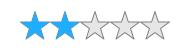# Dealing with Value in UWP Rating (SfRating)

10 May 20212 minutes to read

## Setting the rated value

`Value` property is used to get or set the rate value in `SfRating` control.

``<input:SfRating ItemsCount="5" Value="2" x:Name="rating"/>``
``````rating.ItemsCount = 5;

rating.Value = 2;``````
``````rating.ItemsCount = 5

rating.Value = 2``````## Previewing the rate

`PreviewValue` property is used to get the pointer over value in SfRating control, and this value is displayed as tool tip content.## ValueChanged event

`ValueChanged` event fires whenever the value is changed in rating control. Event arguments contain old value and new value.

``<input:SfRating ItemsCount="5" ValueChanged="rating_ValueChanged"/>``
``````private void rating_ValueChanged(object sender, ValueChangedEventArgs e)

{

}``````
``````Private Sub rating_ValueChanged(ByVal sender As Object, ByVal e As ValueChangedEventArgs)

End Sub``````

`SfRating` can also restrict user from changing the rates in control using the `IsReadOnly` property. But preview value changes when pointer moves over the control.

``<input:SfRating ItemsCount="5" Value="2" IsReadOnly="true" x:Name="rating"/>``
``````rating.ItemsCount = 5;

rating.Value = 2;

``````rating.ItemsCount = 5